These functions are the bayesplot equivalent to ggplot2's ggplot2::theme_set() and friends. They set, get, and update the active theme but only apply them to bayesplots. The current/active theme is automatically applied to every bayesplot you draw.

Use bayesplot_theme_get() to get the current bayesplot theme and bayesplot_theme_set() to set a new theme. bayesplot_theme_update() and bayesplot_theme_replace() are shorthands for changing individual elements.

bayesplot_theme_get()

bayesplot_theme_set(new = theme_default())

bayesplot_theme_update(...)

bayesplot_theme_replace(...)

## Arguments

new The new theme (list of theme elements) to use. This is analogous to the new argument to ggplot2::theme_set(). A named list of theme settings.

## Value

bayesplot_theme_get() returns the current theme. The other three functions (set, update, replace) invisibly return the previous theme so it can be saved and easily restored later. This is the same behavior as the ggplot2 versions of these functions.

## Details

bayesplot_theme_set() and friends only apply to bayesplots. However, ggplot2::theme_set() can also be used to change the bayesplot theme. Currently, setting a theme with ggplot2::theme_set() (other than the ggplot2 default ggplot2::theme_grey()) will override the bayesplot theme.

## See also

theme_default() for the default bayesplot theme.

bayesplot-helpers for a variety of convenience functions, many of which provide shortcuts for tweaking theme elements after creating a plot.

bayesplot-colors to set or view the color scheme used for plotting.

## Examples

library(ggplot2)

# plot using the current value of bayesplot_theme_get()
# (the default is bayesplot::theme_default())
x <- example_mcmc_draws()
mcmc_hist(x)#> stat_bin() using bins = 30. Pick better value with binwidth.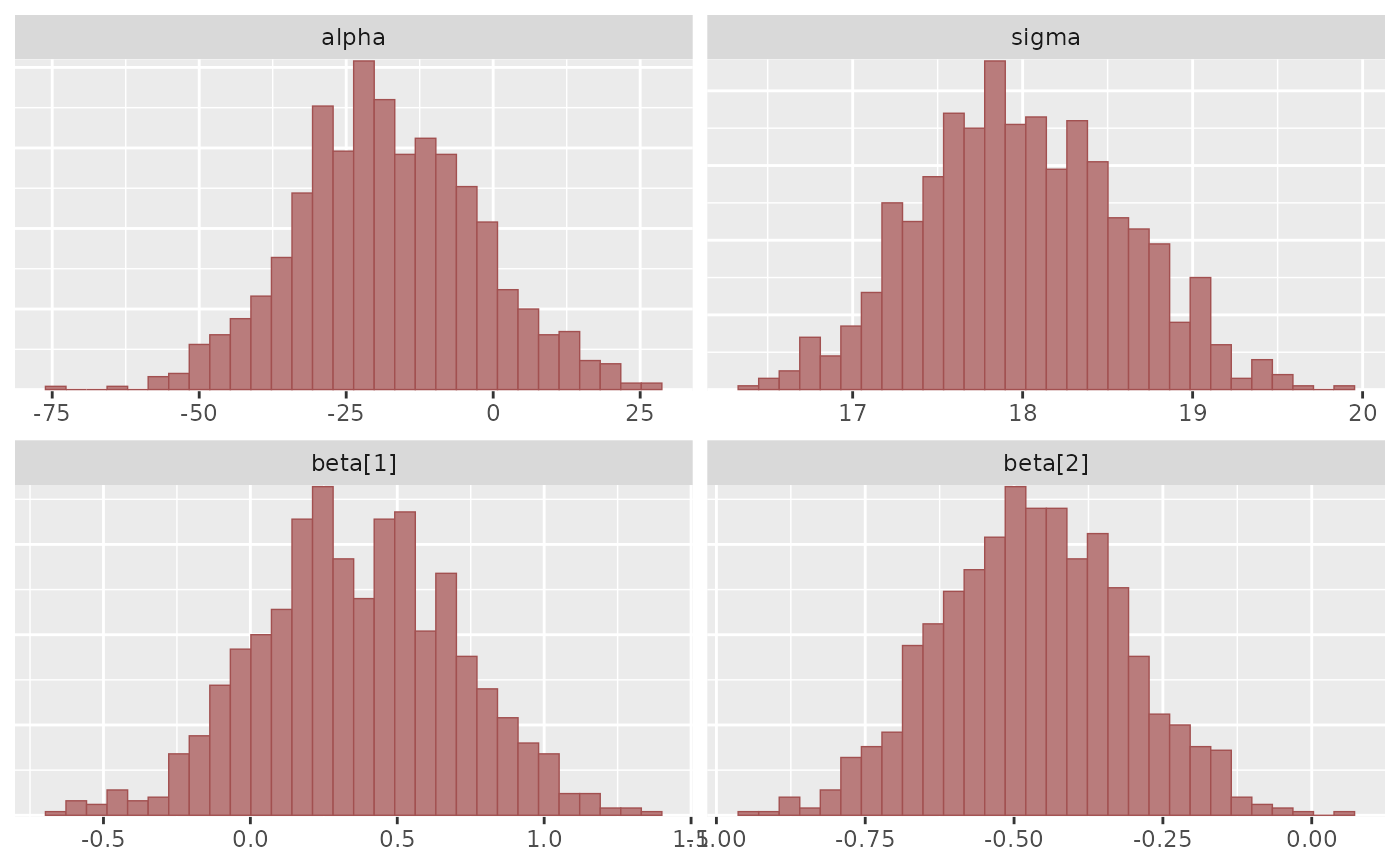# change the bayesplot theme to theme_minimal and save the old theme
old <- bayesplot_theme_set(theme_minimal())
mcmc_hist(x)#> stat_bin() using bins = 30. Pick better value with binwidth.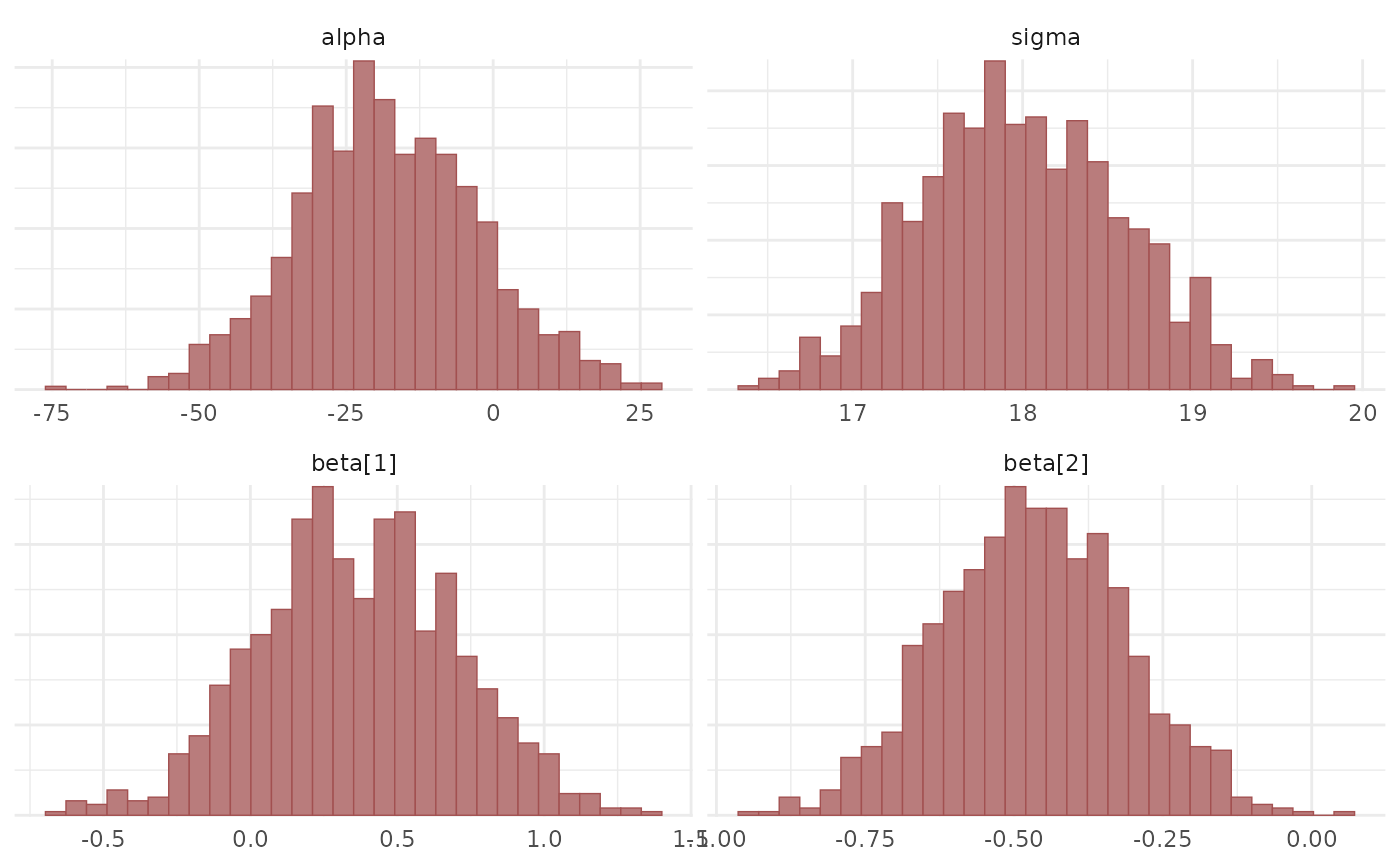# change back to the previous theme
bayesplot_theme_set(old)
mcmc_hist(x)#> stat_bin() using bins = 30. Pick better value with binwidth.# change the default font size and family for bayesplots
bayesplot_theme_update(text = element_text(size = 16, family = "sans"))
mcmc_hist(x)#> stat_bin() using bins = 30. Pick better value with binwidth.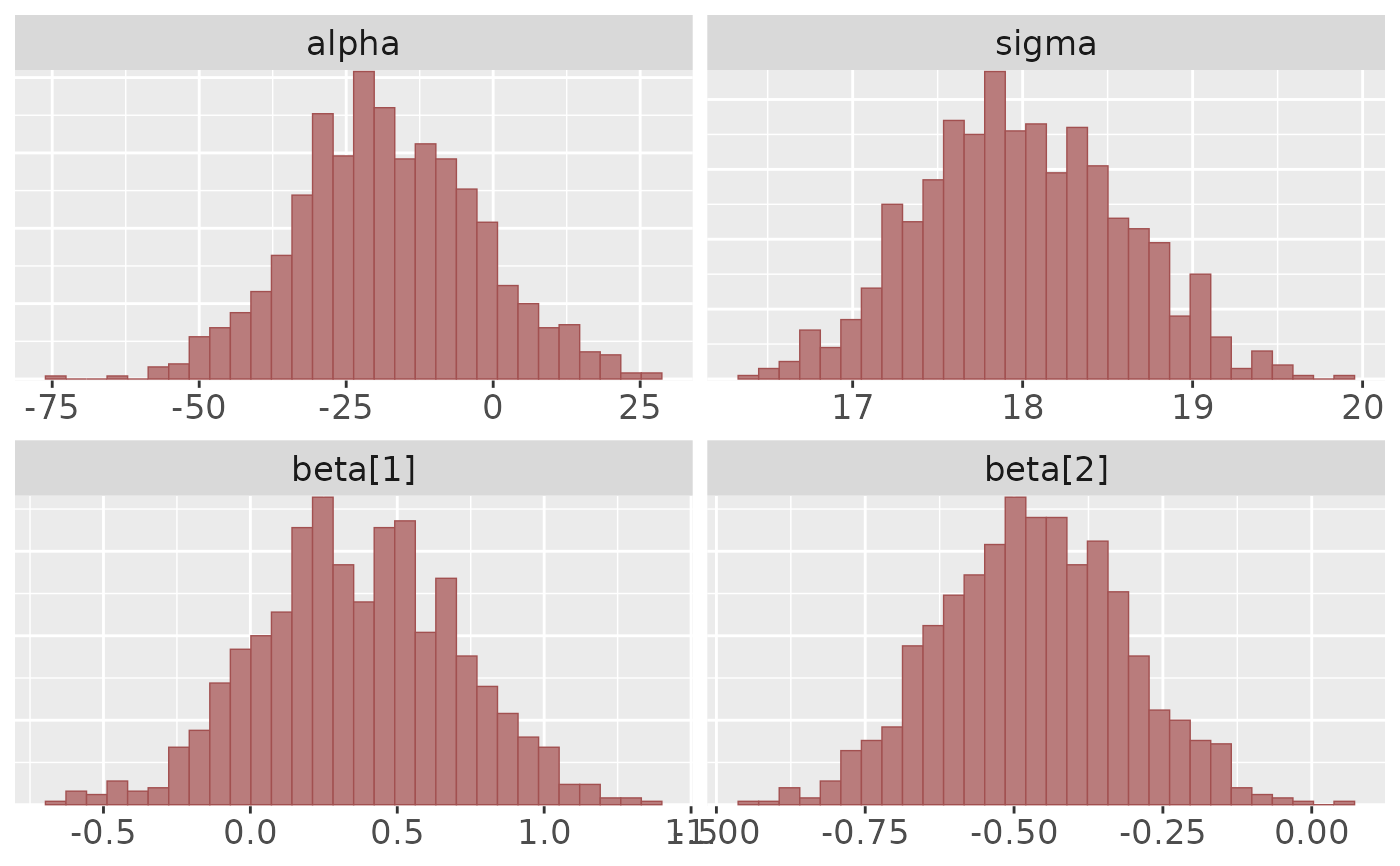# change back to the default
bayesplot_theme_set() # same as bayesplot_theme_set(theme_default())
mcmc_hist(x)#> stat_bin() using bins = 30. Pick better value with binwidth.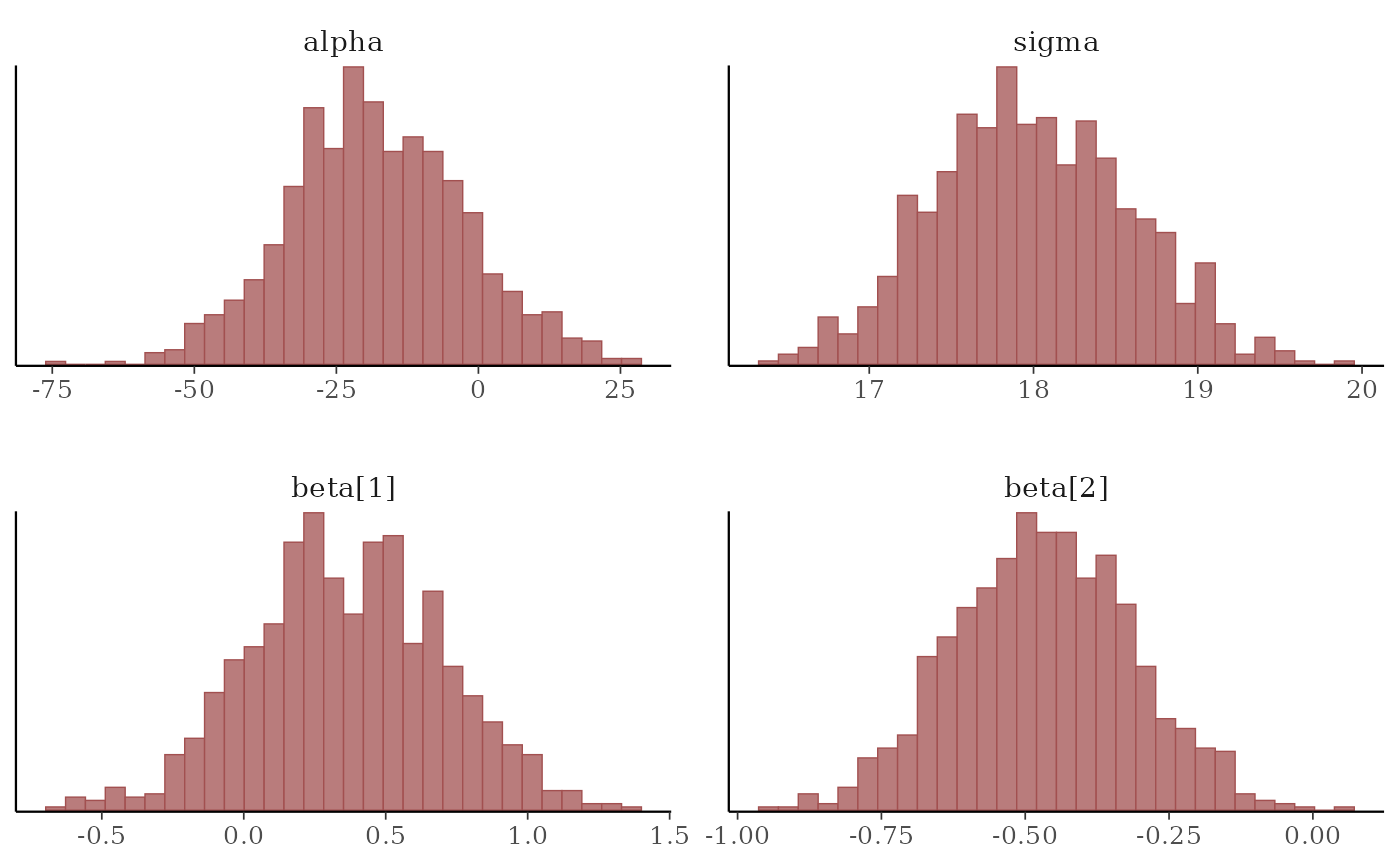# updating theme elements
color_scheme_set("brightblue")
bayesplot_theme_set(theme_dark())
mcmc_hist(x)#> stat_bin() using bins = 30. Pick better value with binwidth.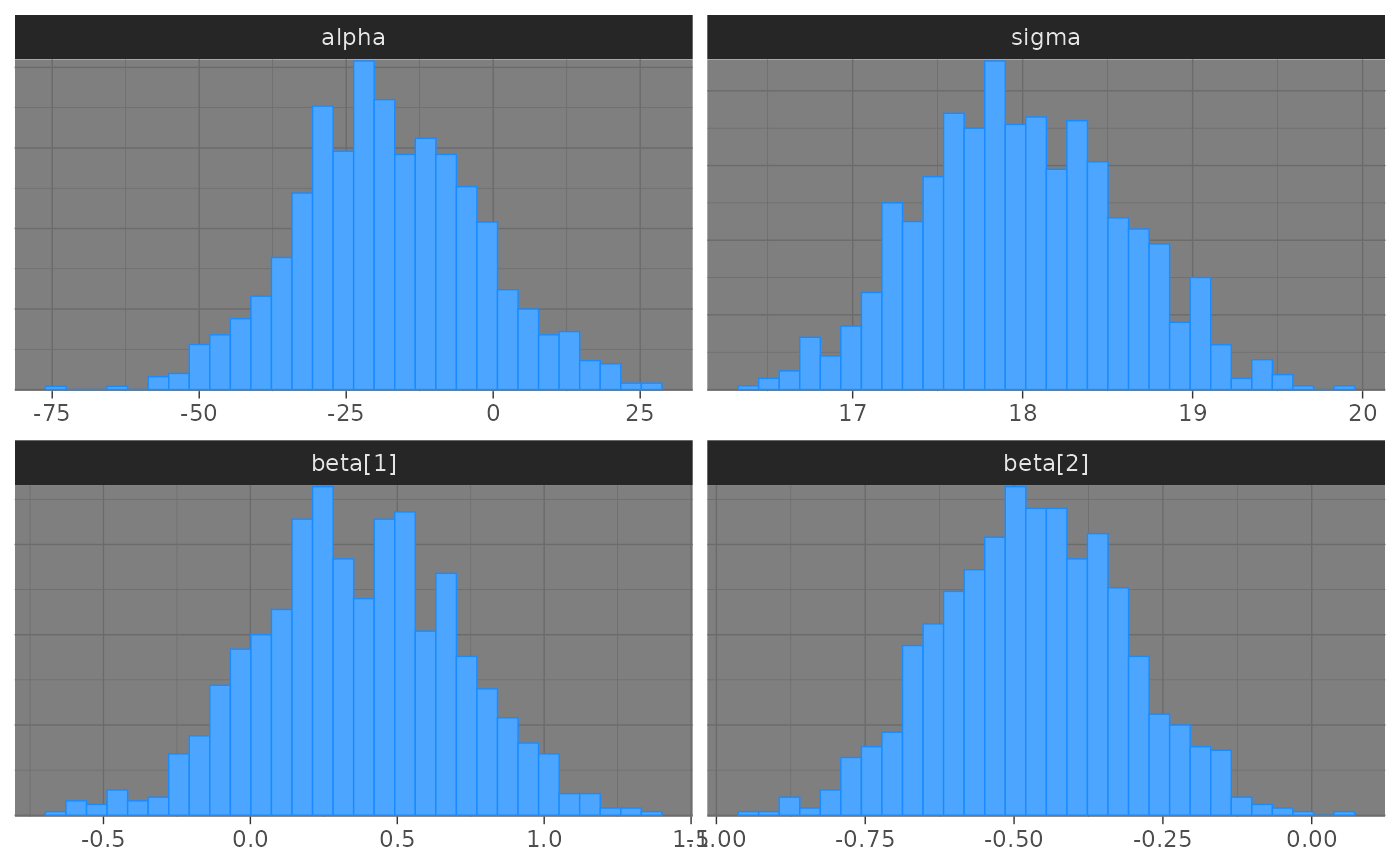bayesplot_theme_update(panel.background = element_rect(fill = "black"))
mcmc_hist(x)#> stat_bin() using bins = 30. Pick better value with binwidth.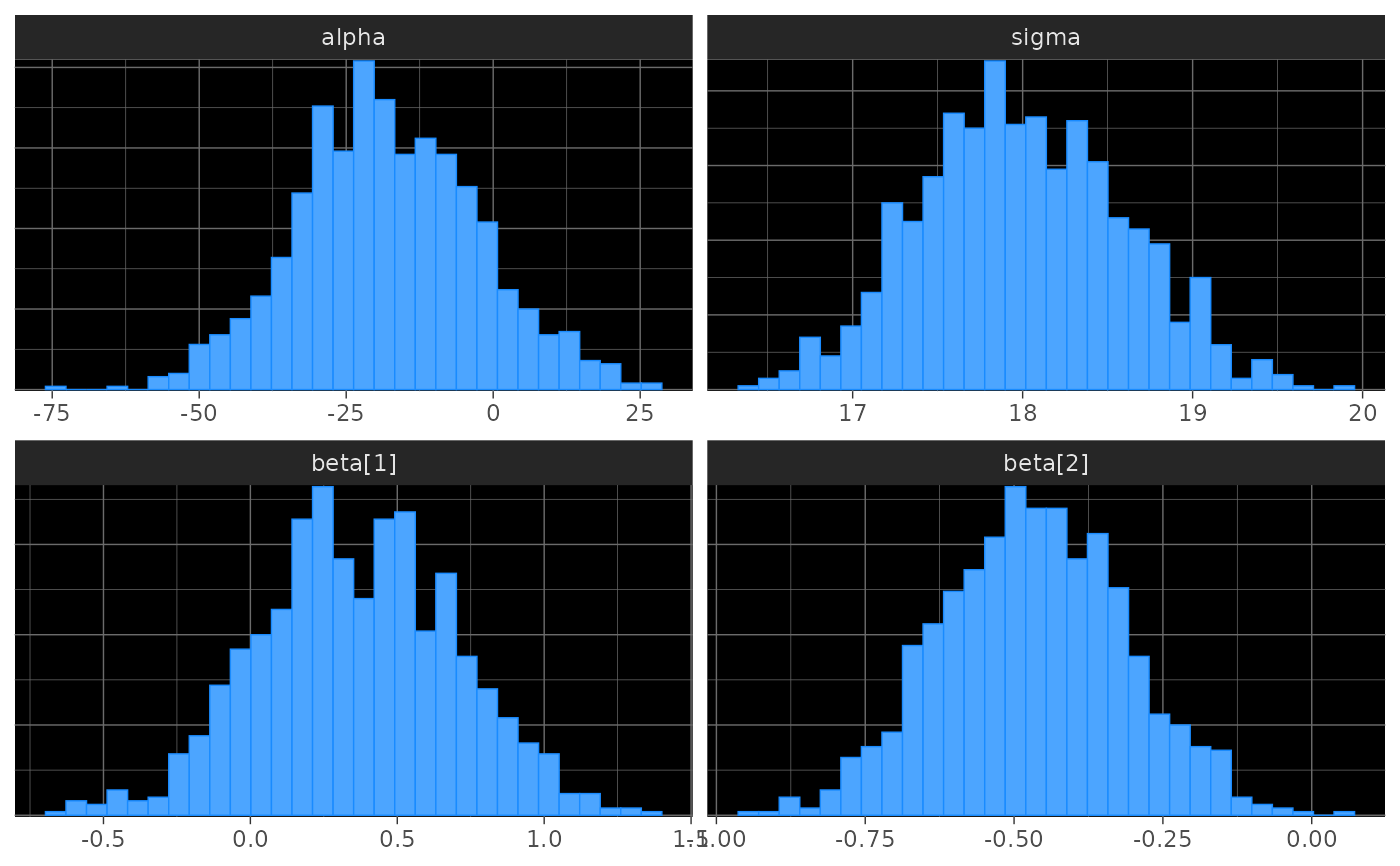# to get the same plot without updating the theme we could also have
# used the bayeplot convenience function panel_bg()
bayesplot_theme_set(theme_dark())
mcmc_hist(x) + panel_bg(fill = "black")#> stat_bin() using bins = 30. Pick better value with binwidth.